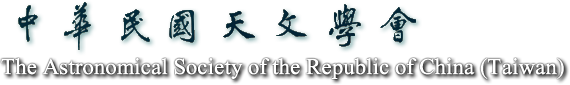2020天文年會

# 論文摘要

## Upper mass stability limit of (co-orbital) horseshoe planets

### [ Oral ]

A. Paula Granados C.; Aaron C. Boley

In this talk, we expand our research on the stability limit of two equal-mass planets in a horseshoe (coorbital) configuration and consider non-circular orbits. Through numerical simulations, we test the long term stability (up to 100 Myrs) of an arrange of two equal-mass planets with planet-to-star mass ratio ranging from 1E-4 to 5E-2 and initial period ratio between 1 and 1.1 (with a ~ 1 au). We also investigate the effect of three different initial eccentricities (i.e., e=0, 0.01 and 0.1) and two initial relative mean longitudes (dl = 60 and 180 deg) on the stability of these systems. Among other parameters, we calculate and analyze the coorbital period of the stable realizations; this period has a functional form which differs between the tadpole and horseshoe configurations, as discussed in this talk. Finally, we find that the upper mass stability limit for horseshoe planets in terms of the central mass, Mc, varies with the eccentricity. Respectively for e=0.0, 0.01 and 0.1, the corresponding stability limits are 2.5E-3 Mc , 1E-3 Mc and 5E-4 Mc, regardless of the initial relative mean longitude.